# The amazing librarian

June 2008

Given an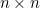matrix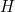and a vector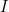of length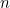, their product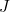is also vector of length(strictly speaking we should writeas a column vector here). The first entry ofis the sum:

 the first entry of the first row of×  the first entry of+ the second entry of the first row of×  the second entry of+ ... + the last entry of the first row of×  the last entry of.

Now recall how we have built the matrix. The first row records the information on back links of the page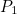: the first entry of this row is zero ifdoes not link to itself and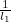if it does, the second entry of the first row is 0 if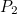does not link toand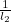if it does, etc. Here the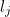are the total number of links on page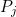. Now each entry of the first row ofis multiplied by the corresponding entry of the importance vector. So the first entry is multiplied by the importance of, to get either 0 or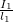. The second entry is multiplied by the importance ofto get either 0 or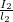, etc. Putting all this together shows that the first entry of the vectoris equal to the sum of the importance of the pages that link to page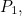weighted by the total number of links on those pages. This is precisely what we defined the importance of pageto be. So the first entry ofis equal to the first entry of the importance vector. The second entry ofis the sum:
 the first entry of the second row of×  the first entry of+ the second entry of the second row of×  the second entry of+ ... + the last entry of the second row of×  the last entry of.

Again we see that the second entry ofis equal to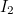, the second entry of the importance vector. The multiplication carries on correspondingly for each row of, and this shows that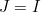.